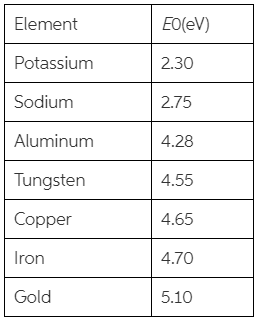# Problem: A) Which metals exhibit the photoelectric effect for light with λ  = 400nm?A. sodiumB. copperC. goldB) Which metals exhibit the photoelectric effect for light with λ =250 nm?A. goldB. ironRefer to the table below.

###### FREE Expert Solution

The energy of a quantised light is expressed as:

$\overline{){\mathbf{E}}{\mathbf{=}}\frac{\mathbf{h}\mathbf{c}}{\mathbf{\lambda }}}$, where h is the Plank's constant and c is the speed of light in a vacuum.

94% (369 ratings)###### Problem Details

A) Which metals exhibit the photoelectric effect for light with λ  = 400nm?

A. sodium

B. copper

C. gold

B) Which metals exhibit the photoelectric effect for light with λ =250 nm?

A. gold

B. iron

Refer to the table below.